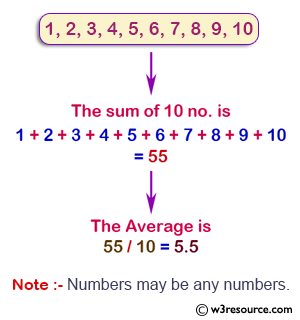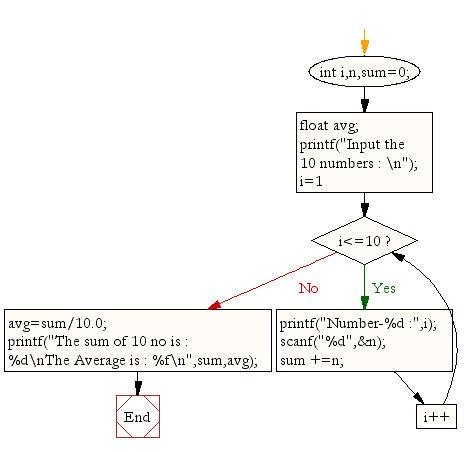﻿ C Program: Read 10 numbers and find their sum and average - w3resource# C Exercises: Read 10 numbers and find their sum and average

## C For Loop: Exercise-4 with Solution

Write a program in C to read 10 numbers from keyboard and find their sum and average.

Pictorial Presentation:Sample Solution:

C Code:

``````#include <stdio.h>
void main()
{
int i,n,sum=0;
float avg;
printf("Input the 10 numbers : \n");
for (i=1;i<=10;i++)
{
printf("Number-%d :",i);

scanf("%d",&n);
sum +=n;
}
avg=sum/10.0;
printf("The sum of 10 no is : %d\nThe Average is : %f\n",sum,avg);

}
```
```

Sample Output:

```Input the 10 numbers :
Number-1 :1
Number-2 :2
Number-3 :3
Number-4 :4
Number-5 :5
Number-6 :6
Number-7 :7
Number-8 :8
Number-9 :9
Number-10 :10
The sum of 10 no is : 55
The Average is : 5.500000
```

Flowchart:C Programming Code Editor:

Improve this sample solution and post your code through Disqus.

What is the difficulty level of this exercise?

Test your Programming skills with w3resource's quiz.

﻿